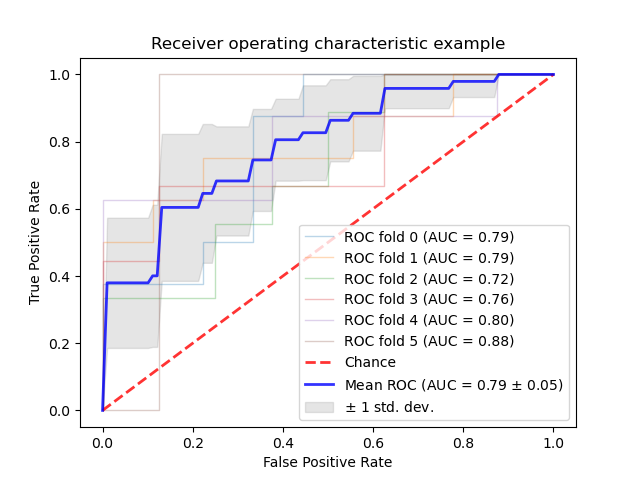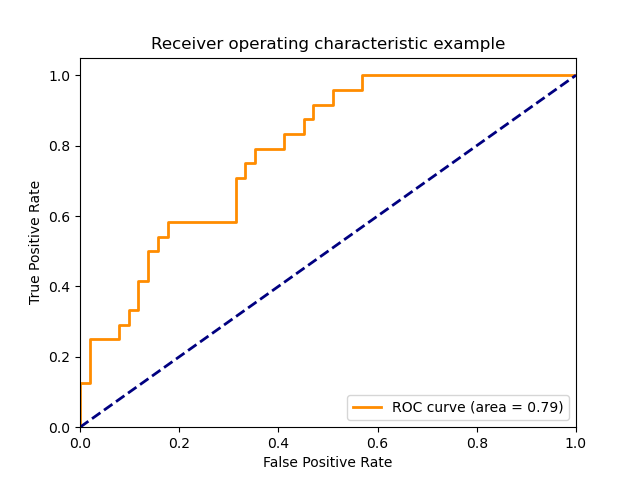# sklearn.metrics.roc_auc_score¶

sklearn.metrics.roc_auc_score(y_true, y_score, *, average='macro', sample_weight=None, max_fpr=None, multi_class='raise', labels=None)

y_true array-like of shape (n_samples,) or (n_samples, n_classes)

y_score array-like of shape (n_samples,) or (n_samples, n_classes)

average {‘micro’, ‘macro’, ‘samples’, ‘weighted’} or None, default=’macro’

- 'micro':

- 'macro':

- 'weighted':

- 'samples':

sample_weight array-like of shape (n_samples,), default=None

max_fpr float > 0 and <= 1, default=None

multi_class {‘raise’, ‘ovr’, ‘ovo’}, default=’raise’

- 'ovr':

- 'ovo':

labels array-like of shape (n_classes,), default=None

auc float

average_precision_score

precision-recall曲线下的面积

roc_curve

3 Provost, F., Domingos, P. (2000). Well-trained PETs: Improving probability estimation trees (Section 6.2), CeDER Working Paper #IS-00-04, Stern School of Business, New York University.

>>> import numpy as np>>> from sklearn.metrics import roc_auc_score>>> y_true = np.array([0, 0, 1, 1])>>> y_scores = np.array([0.1, 0.4, 0.35, 0.8])>>> roc_auc_score(y_true, y_scores)0.75

## sklearn.metrics.roc_auc_score应用示例¶